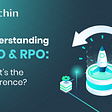# embbed function simulink with signal

Hi, I am trying to simulate the signal by Embedded matlab function in sumulink. The function has two input T=10e-6,B=30e6 and one output. The code as below

function y = chirp_gen1(T,B) %#codegen coder.extrinsic(‘plot’);

K=B/T; %chirp slope Fs=10*B;Ts=1/Fs; %sampling frequency and sample spacing N=T/Ts; t=linspace(-T/2,T/2,N); y=exp(1j*pi*K*t.²); %chirp signal %y=real(St);

plot(t*1e6,real(y),’-+’);

If I run in matlab editor the program works fine. But if I run in simulink, it alway shows N is not constant “The number of points N must be a constant”, t is “Undefined function or variable ‘t’. The first assignment to a local variable determines its class”. So I went to change N =1000, the function will plot the signal. But if I connect to the scope. It does not show the signal as it is. I think some things relate to continuous of ‘t’. So, What wrong with the code above and how to get the output ‘y’ can connect to the scope in simulink block?

Thanks

Matlabsolutions.com provide latest MatLab Homework Help,MatLab Assignment Help for students, engineers and researchers in Multiple Branches like ECE, EEE, CSE, Mechanical, Civil with 100% output.Matlab Code for B.E, B.Tech,M.E,M.Tech, Ph.D. Scholars with 100% privacy guaranteed. Get MATLAB projects with source code for your learning and research.

I was able to make your example work in Simulink after adding the following line before call to “linspace”:

`assert(N<10000);`

Basically, this line tells Simulink that the value of N cannot be greater than the given maximum (10000), and so linspace call knows how much memory to allocate for its internal computation. In Simulink, linspace (and all other functions used in MATLAB Function block) need to know the maximum size of their internal data, and they get it by looking at the maximum values of their inputs.

Of course, you should change 10000 to whatever is the right maximum value for N in your example, depending on your inputs.

--

--

--

## More from Technical Source

Simple! That is me, a simple person. I am passionate about knowledge and reading. That’s why I have decided to write and share a bit of my life and thoughts to.

Love podcasts or audiobooks? Learn on the go with our new app.

## Longest Consecutive Sequence — Day 29(Python)## Becoming a Data Engineer: Resume Writing Tips## Zero to One in Python: Part-2 -Actually Getting started## Understanding RTO and RPO: What’s the Difference?## 🎯The more points you score, the higher the multiplier for the chance of obtaining keys will be!🔑🔑## Domain Oriented Data Architecture — Data Mesh## Technical Source

Simple! That is me, a simple person. I am passionate about knowledge and reading. That’s why I have decided to write and share a bit of my life and thoughts to.

## Goals, Pageview and Custom Parameters## Introducing Eric: Senior Data Engineer